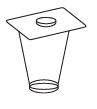Courses

# Force And Laws Of Motion, Science, Class 9 - Test

## 10 Questions MCQ Test Class 9 Science by VP Classes | Force And Laws Of Motion, Science, Class 9 - Test

Description
This mock test of Force And Laws Of Motion, Science, Class 9 - Test for Class 9 helps you for every Class 9 entrance exam. This contains 10 Multiple Choice Questions for Class 9 Force And Laws Of Motion, Science, Class 9 - Test (mcq) to study with solutions a complete question bank. The solved questions answers in this Force And Laws Of Motion, Science, Class 9 - Test quiz give you a good mix of easy questions and tough questions. Class 9 students definitely take this Force And Laws Of Motion, Science, Class 9 - Test exercise for a better result in the exam. You can find other Force And Laws Of Motion, Science, Class 9 - Test extra questions, long questions & short questions for Class 9 on EduRev as well by searching above.
QUESTION: 1

Solution:
QUESTION: 2

Solution:
QUESTION: 3

### The rate of change of momentum of an object is proportional to

Solution: Acceleration can be non- zero only if there is a net external force on the body. The rate of change of momentum of a body is directly proportional to the applied force and takes place in the direction in which the force acts. To every action, there is always an equal and opposite reaction.
QUESTION: 4

If two balls of different masses are dropped on sand, the depths of penetration is same if:

Solution:
QUESTION: 5

The coin remains at rest in the figure shown. This is due toSolution:
QUESTION: 6

A force of 50 N moves a body.

Solution:
QUESTION: 7

Fielder giving a swing while catching a ball is an example of

Solution:

When you swing your hand, it will take more time to stop the ball. Hence the rate of change of momentum of the ball will decrease. So force acting on your hand will be less.

QUESTION: 8

Action and reaction forces

Solution:
QUESTION: 9

When we stop pedaling the bicycle it stops because

Solution:
QUESTION: 10

A football and a stone has same mass

Solution: